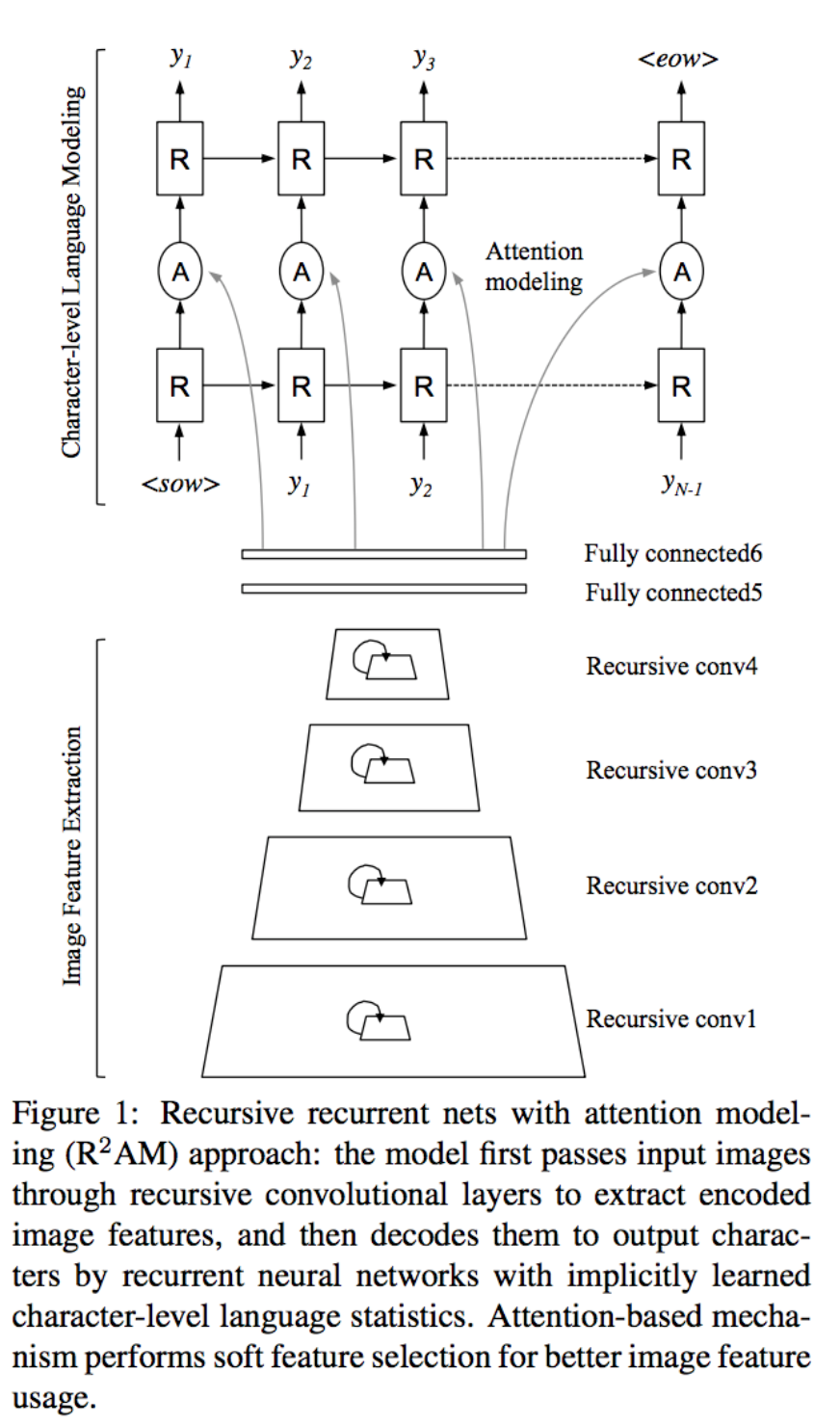Deepmind’s end-to-end text spotting pipeline using CNN

Image in this section is taken from Source Max Jaderberg et al unless stated otherwise.

Proposal generations

First, it uses cheap classifiers to produce high recall region proposals but not necessary with high precision. Later, it introduces methods to reject more false positives. A proposal is consider a true positive if it overlaps with the true value by a threshold value:

$\frac{b_p \cap b_t }{b_p \cup b_t} > threshold$

It uses a threshold as low as 50% which may generates many false positive.

• Use both edge boxes to give proposals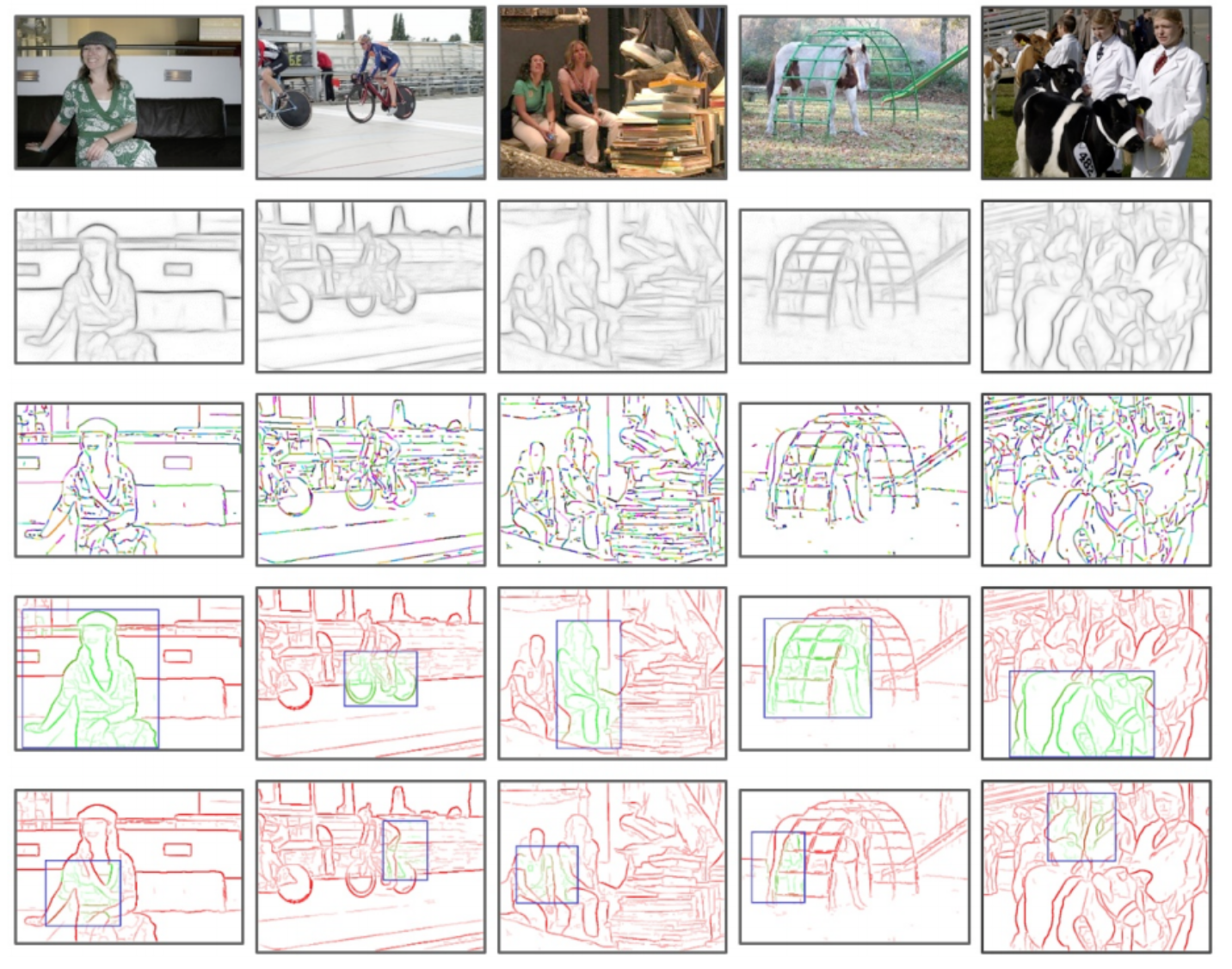Source Lawrence Zitnick et al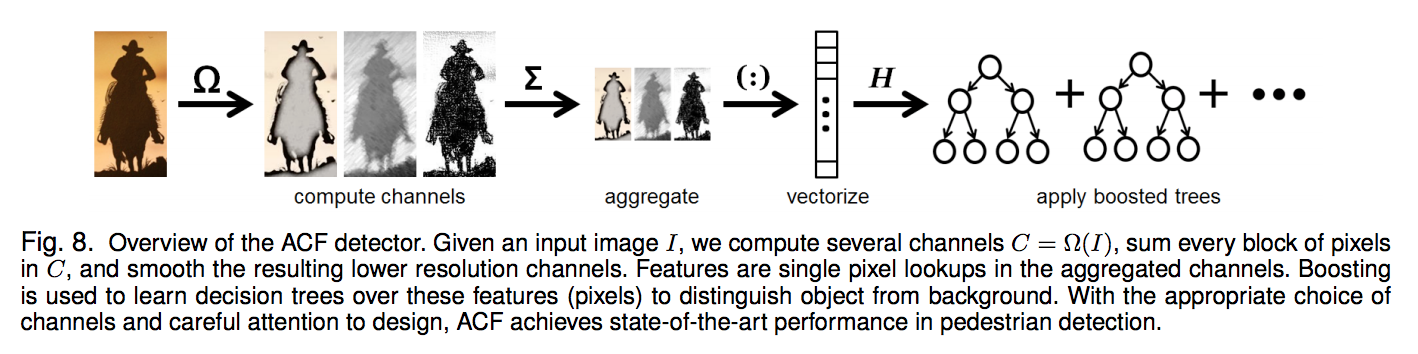Filter and refinement

Thousands of bounding boxes are generated in the proposal generations which many are false-positive. It uses

• a random forest classifier on HOG features to eliminate false positive.
• and a bounding box regressor using CNN to refine the boundary box (from red to green).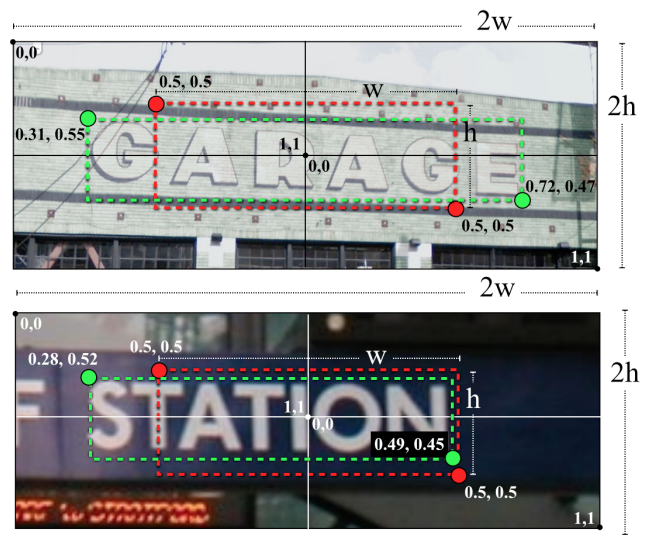Text Recognition

Generate training data: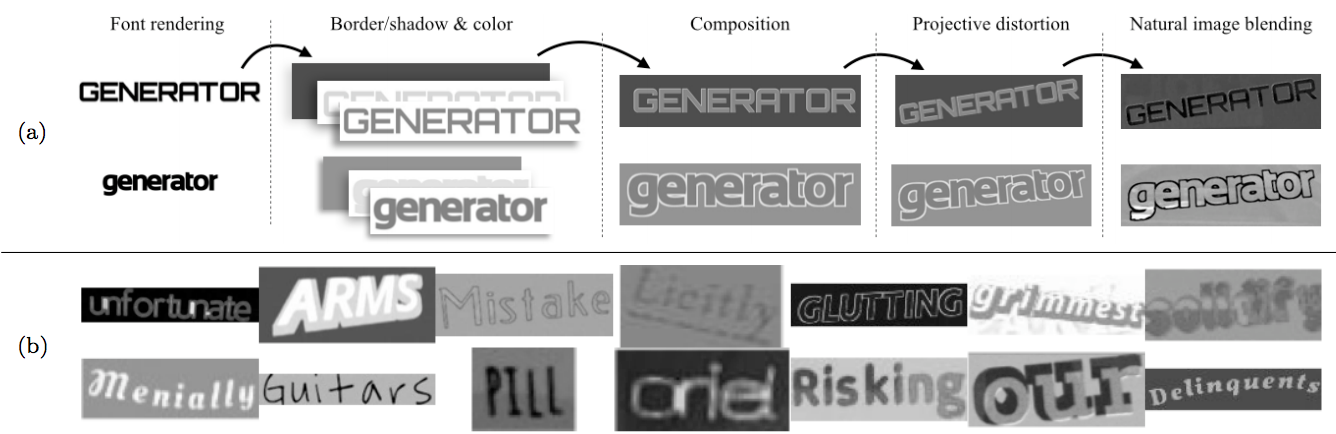CNN classifier:

$\begin{split} w^{∗} & = \arg \max_{w∈W} P(w \vert x,L) \quad \text{which L is the language}\\ P(w \vert x,L) &= \frac{P(w \vert x)P(w \vert L)P(x)}{P(x \vert L)P(w)} \\ &= \frac{P(w \vert x)P(w \vert L)}{P(w)} \quad \text{given x is independent of L}\\ w^{∗} & = \arg \max_{w∈W} P(w \vert x)P(w \vert L) \\ \end{split}$

$$P(w \vert x)$$ is modelled by the softmax output of CNN by resample the region to a fixed height and width.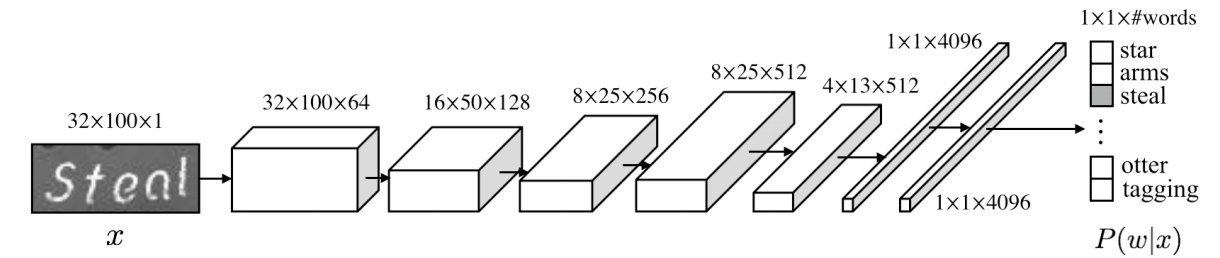and the language based word prior $$P(w \vert L)$$ can be modelled by a lexicon.

Merging & ranking

It may still contain false positives and duplicates, so a final merging and ranking of detections is done. (text spotting)

For each bonding box:

$\begin{split} w_b & = \arg \max_{w∈W} P(w \vert b, I) \\ s_b &= \max_{w∈W} P(w \vert b, I) \\ \end{split}$

To merge the detections of the same word, it applies a non maximum suppression (NMS) on detections with the same word label. It also performs NMS to suppress non-maximal detections of different words with some overlap.

It performs multiple rounds of bounding box regression and NMS to refine the bounding box. Performing NMS between each regression causes similar bounding boxes to be grouped as a single detection. This causes the overlap of detections to converge on a more accurate detection.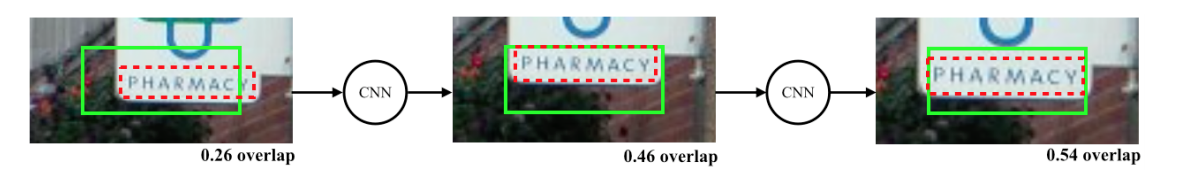Dropbox OCR

Image in this section is from here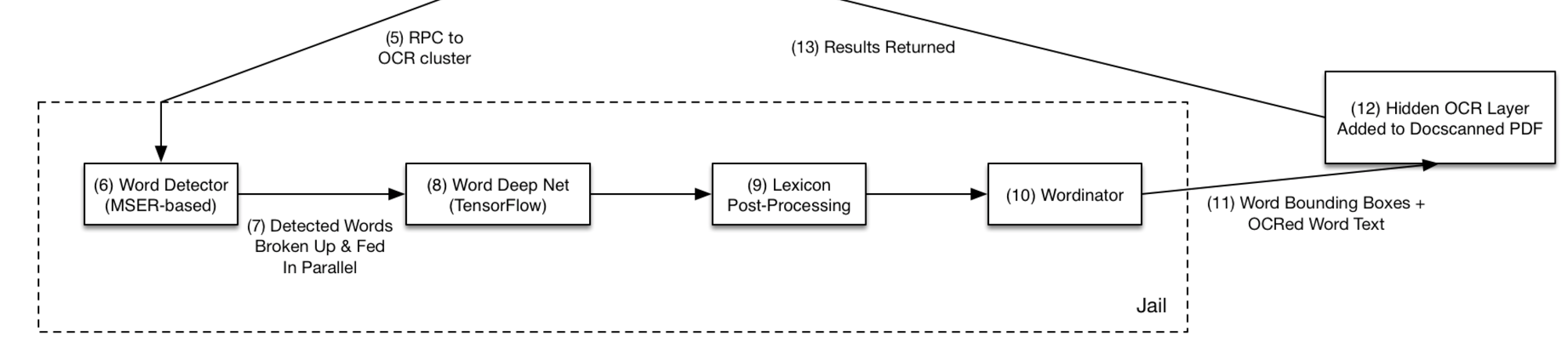Word detector

Use Maximally stable extremal regions in OpenCV for word detector.

Word deep net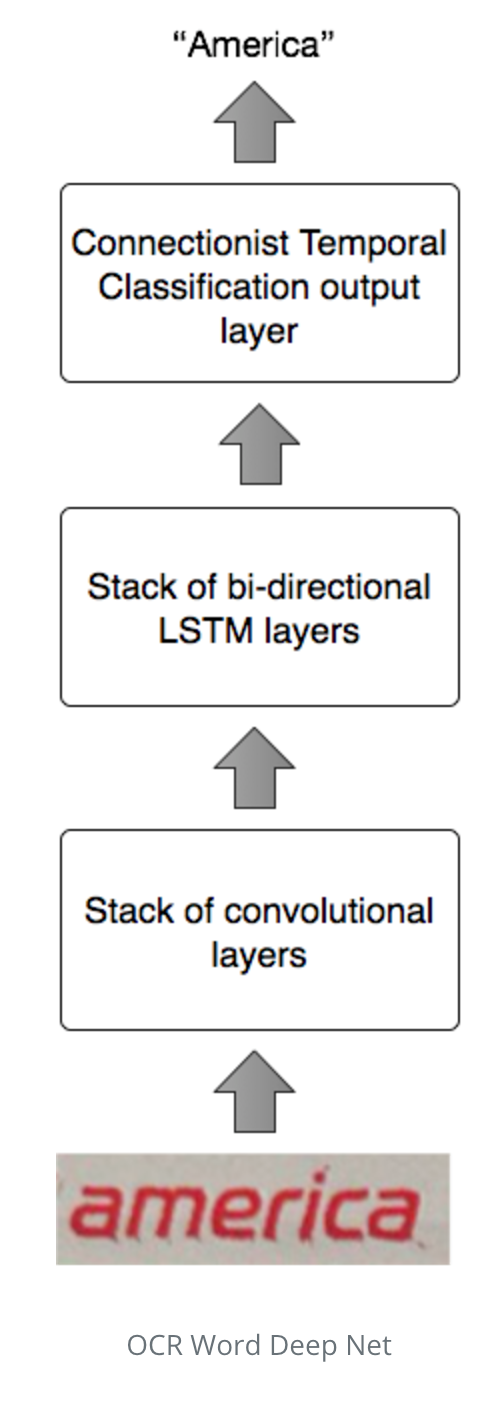If the score was somewhere in the middle, it runs through a lexicon generated from the Oxford English Dictionary, applying different transformations between and within word prediction boxes, attempting to combine words or split them using the lexicon.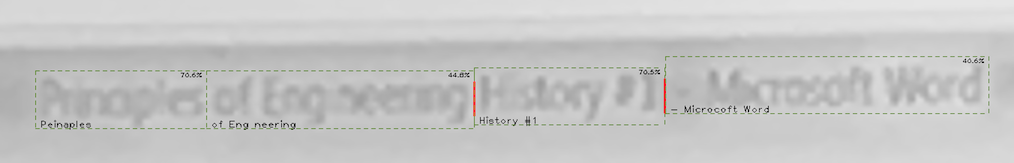Recursive Recurrent Nets with Attention Modeling for OCR

Recursive Recurrent Nets with Attention Modeling for OCR in the Wild, Chen-Yu Lee